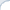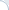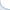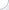## On an inconsistency in IBM Watson papers

More commonly, factoids are extracted using a simple information retrieval-based algorithm. To this end, a question is superficially transformed and is subsequently fed as a query to an information retrieval engine. The result of such a query is a set of passages or documents which match the query well. Then, we extract entities (e.g., person or location names) from text snippets or document titles and finally rank them. One of the most useful ranking features is an answer type. For example, an answer to the question "What is the capital of the USA" is a location, so that we can ignore (or downweigh) entities that are not locations. Another common feature is a popularity (i.e., how often an entity appears in search results). The true answer tends to co-locate with query words, more frequently than unrelated entities.

The retrieval-based approach works pretty well, unless there is a substantial mismatch between question words and words that appear in the text. Query reformulation and expansion may sometime help to overcome the vocabulary mismatch. Yet, this is not always possible, because adding synonyms improves recall at the expense of precision. Consider the following Jeopardy! question: "Unlike most sea animals, in the Sea Horse this pair of sense organs can move independently of one another" (answer eyes). As argued in the paper "Finding needles in the haystack: Search and candidate generation", it is quite hard to find an answer using standard retrieval techniques, because the question mentions an obscure fact. In that, it is much easier to lift the veil of obscurity by checking knowledge base entries related to "sense organs". The IBM Watson creators call this type of search as the PRISMATIC search (named after the knowledge base PRISMATIC).

Further, they say that the PRISMATIC search is both precise and has a good coverage:
"On a test set of 3,508 Jeopardy! questions, the PRISMATIC candidate generator produces answers for 42.6% of them (1,495 questions). ... In addition, the PRISMATIC candidate generator produces far fewer wrong candidate answers than other candidate generation techniques. On average, 1 out of 57 PRISMATIC candidates is the correct answer to a given question, compared with 1 out of 134 candidates from the rest of the candidate generation pipeline."

One may interpret the previous statement as follows: The binary recall of this answer-generation technique is close to 50%. That is, for roughly half of the questions, the PRISMATIC search generates a short list of answers, one of which is the correct one! If this were true, it would have been great news for all QA folks. However, in another paper "Finding needles in the haystack: Search and candidate generation" creators of Watson present a more systematic evaluation of the PRISMATIC search. They find that a related binary recall (see Table 1) is only 8%. In other words, this strategy is potentially useful only for about one question out of ten.

It is a bit unclear as to how reconcile both statements. One good suggestion came from Petr Baudis. Petr proposed the following explanation: When authors say that the PRISMATIC candidate generator produces answers for 42.6% of all questions, this does not necessarily mean "42.6% of questions for which we get at least one correct answer on the candidate list". It may simply mean that in 42.6% of all cases, the PRISMATIC produces some answers, but, as we learn from another paper, they are mostly incorrect. The binary recall of 8% is still substantially better than the binary recall of the answer lookup (which is 3.5%, see Table 1), but much lower than that of the retrieval-based approach (which is almost 80%).

To conclude, the PRISMATIC search appears to be a good complementary strategy that fits somewhere between the classic retrieval-based approach and the exact answer lookup. Yet, it is not a replacement for the classic information retrieval-based approach.

## When average precision is equal to recall

In certain situations, an average precision is equal to recall. Proving that this is the case and finding ways to deal with this issue can be a good problem for an IR course homework and might help students to grok the notion of the average precision. This problem was inspired by the real research paper where authors used the mean average precision without realizing it was equal to recall.

Imagine that a nearest-neighbor search should retrieve 10 images most similar to a query image. The search is typically not ideal and some found images would be quite different from the query image. If we make humans judge retrieved results, it can be possible to evaluate search effectiveness via the (mean) average precision. If human judgments are not available, one may decide to use a proxy metric: An image is relevant if it is a true nearest neighbor, i.e., it is one of the 10 most closest images with respect to the values of the distance function. An exact search method always returns the true 10 nearest neighbors, but an approximate method may fail to do so. A degree of "sloppiness" can be estimated using the average precision, right?

I think that this wrong and here is why. In this formulation, a retrieved image is relevant when a distance from this image to the query object does not exceed the distance from the query image to the 10-th true nearest neighbor. Furthermore, all returned objects are normally sorted in the order of increasing distance from the query. Consequently, there will be $R\le10$ objects with distances not exceeding the distance to the 10-th nearest neighbor. And these images will clearly be considered "relevant". The following $10-R$ images will have distances larger than the distance to the 10-th nearest image and, thus, they will not be considered relevant.

It is not hard to see that in this case the average precision is equal to $R/10$ (by the definition of the average precision, P@N is one if $N \le R$ and is zero otherwise). However, the recall (the fraction of true nearest neighbors returned) is also $R/10$.

A good homework problem can ask the following:

1) To prove the equality of the average precision and the recall;
2) To describe ways of dealing with this issue, i.e., can we still use the average precision (after some fixes)? If not, what else can be used?

The second question is clearly open-ended, because (in addition to introducing real human judgments) multiple solutions are possible, which include but are not limited to using a rank approximation error.

## Neat code to compare floating-point numbers

Floating point arithmetic is not exact and not even deterministic. Not only results may be different across platforms and compilers, but in a non-strict (but fast) mode an outcome of the same operation may depend on the invocation context. If you use an aggressively optimizing compiler (such as Intel), it is silly to assume that same function arguments will always produce same results. The outcomes will be the same in many cases, but the results might also fluctuate by a few units in the last place (ULP). Code written with the assumption that results are always the same (i.e., assuming that floating point arithmetic is always consistent) may contain bugs that are hard to reproduce and fix.

To avoid such bugs, we may need to compare floating points numbers approximate rather than exactly. So, how do we do this? It is apparently easy to come up with a simple comparison code that works in most cases, but likely to fail in some rare cases. After some googling, I found a neat implementation of the Bruce Dawson algorithm from "Comparing Floating Point Numbers". The implementation was buried in the Google C++ Test Framework (authors Zhanyong Wan and Sean Mcafee). Thanks to Fred Richards, it was extracted and repackaged (see Fred's blog entry for a detailed description of the algorithm).

Fred's version was a bit inflexible and included only the hard coded threshold value (for numbers to to be considered the same). Thus, I had slightly modified the code to accept a threshold value as an argument of the comparing function. In addition, I made the ULP_diff function publicly available and improved the testing example. If you need a code to compare floating point numbers approximately, you can grab it from my repository.

UPDATE: I forgot to mention that Boost does have the code with similar functionality. Yet, I was looking for the code without Boost dependencies.

## What's wrong with _mm_extract_ps and _mm_extract_pd?

If you are programming in C/C++, you can use Single-Instruction Multiple Data(SIMD) commands. The beauty of these commands is that they operate on small vectors rather than singular scalar values. For example, we can multiply or subtract 4 pairs of floating point numbers at the same time. SIMD commands are available on many CPUs, but my post is Intel-specific.

The current standard set of instructions on Intel SSE 4 supports vector operations on 128-bit data elements. The vector size in bits is always 128, but the size of data elements is different. You can operate on sixteen 8-bit values, eight 16-bit values, four 32-bit values, and two 64-bit values. The type of data elements can also be different. A 32-bit value, e.g., can be an integer or a single-precision floating point number. A 64-bit value can be an integer, or a double-precision floating value. Longer, e.g., 256-bit vectors are also supported by newer CPUs, but I do not consider them here.

And it is kinda nightmarish, because you cannot use your regular '+', '-', '*' any more. You have a separate function for each data type (and, even worse, a bunch of conversion functions). For instance, addition of two 4-element floating point vectors is _mm_add_ps, addition of two 2-element double-precision floating point vectors is _mm_add_pd, and addition of two 4-element integer vectors is _mm_add_epi32

It is bad, but not terribly bad, because there is a naming convention that helps you navigate through this jungle. As you might have noticed, all operations start with the same prefix _mm_, then there is a part indicating the type of the operation, and, finally, a type-specific suffix. These suffixes are as follows:

epi8 for 8-bit integers;
epi16 for 16-bit integers;
epi32 for 32-bit integers;
ps for single-precision floating point numbers;
pd for double-precision floating point numbers.

To operate on 128-bit vectors, the CPU uses special 128-bit registers. If you need to extract specific vector elements and store them in regular 32-bit or 64-bit registers, you have to use a special CPU command. Of course, you can always copy vector values to the memory and read back only a necessary portion, but this is rather slow. This is why there are commands that can copy specific elements of a 128-bit vector to a 32-bit or 64-bit CPU register. BTW, store and load operations also follow a convention. The store command for four-element single-precision floating point vectors is _mm_storeu_ps (u in storeu denotes an unaligned write).

The command _mm_exctract_epi8 treats a 128-bit register as a 16-element integer vector. It allows one to extract any of the sixteen integer vector elements (each has a size of 8 bit). _mm_extract_epi16 gives you one of the eight 16-bit vector elements_mm_extract_epi32 extracts one of the four 32-bit integer values. Ok, what does _mm_extract_ps do? Extracts one of the four single-precision floating point numbers, right? Wrong, it also extracts one of the four 32-bit integers. Furthermore, there is no function _mm_extract_pd!

To efficiently extract floating point numbers you need to use functions _mm_cvtss_f32 and _mm_cvtsd_f64. They extract only the first floating point number from the vector. Yet, there is a command to move an arbitrary element of the four-element vector to the first position. This command is called a shuffle instruction. Thus, you can first shuffle an arbitrary element to the first position, and then extract the first element. The name of the shuffle command is a bit of misnomer itself, because shuffle usually means rearranging. Yet, shuffling on Intel is IMHO multiplexing.

It does not bother me much that the actual floating-point extraction functions are missing. Yet, I cannot understand why there is a function _mm_extract_ps with a misleading name and redundant functionality? Anyway, after reading some material on the Web, I have created two simple macros: one for extraction of single-precision and and another for extraction of double-precision floating point numbers. My code is freely available.

## Pages